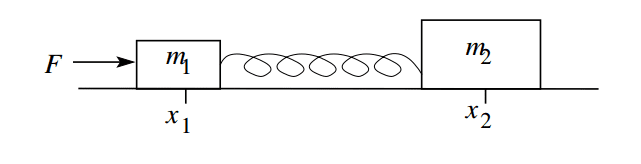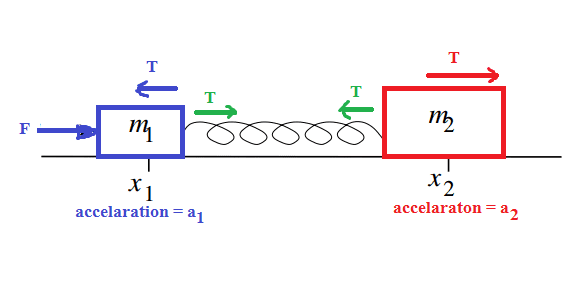# Problem on a mass-spring system

arpon

## Homework StatementSuppose the surface is completely frictionless. Will the spring experience any length change?[/B]

## The Attempt at a Solution

To change the length of the spring, force should be applied from both ends. In this case, there is no force of friction. So, my opinion is, there will be no change of length of the spring.[/B]

Homework Helper
Miss the relevant equation. If that would be something simple like F = m a , then: just suppose for a momoent that you're right. In that case F = m1 a , so m1 undergoes acceleration a = m1/F but there is no force on m2 so m2 stays in place. Somehow I think that's not a sutainable solution. Do you agree ?

arpon
Miss the relevant equation. If that would be something simple like F = m a , then: just suppose for a momoent that you're right. In that case F = m1 a , so m1 undergoes acceleration a = m1/F but there is no force on m2 so m2 stays in place. Somehow I think that's not a sutainable solution. Do you agree ?
I thought, the force will be applied on the whole system, then , ##a = F/(m_1 + m_2)##

Basic physics: $F=m \cdot a$. Since the total mass pushed by F is m1+m2 (plus the mass of the spring, ignored here), this gives you a value for a. Now look at m2. You know a, calculate the force F2 on m2. Since the spring is fastened to m2, an opposite and equal force is applied to the spring. Since we ignore the mass of the spring, the same force will act on the other end of the spring (but in the opposite direction). Will the spring change its length?

arponSo, it is, I think:
for ##m_1## :
##F-T = m_1 a_1##
for ##m_2## :
##T = m_2 a_2##
the tension on the spring is ##T## ;

Homework Helper
In fact, the right answer to the question in the exercise is: "it depends".
Namely on what exactly the figure represents.
There is one situation imaginable where the spring does not change length: if the figure represents a situation where both m1 and m2 undergo an acceleration a0 = F / (m1+ m2) and the spring is at equilibrium. In that one particular case the spring length can remain constant. This is highly artificial. All other cases: no.

The most likely interpretation of the figure is: this is a picture at time t = 0 of m1 and m2 at rest and the spring at equilibrium length, not applying any force. At t=0 a constant force F is applied on m1. If that is the right description, then read on, but if that is wrong, correct me.

I agree with Svein to some extent (the total mass pushed by F is m1+m2), but not much further. The a from F / (m1+ m2) is not the a at t=0 for m1 and also not the a at t=0 for m2. What do you think the instantaneous acceleration at t=0 for m1 is ? and for m2 ?

crossed post #5. I agree with that post.
We need an equation for T. Any ideas ?